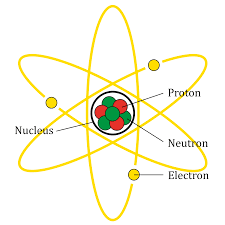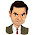# Why the atom song have lyricsThe way the planets revolve around the Sun, we all have seen the left picture showing the orbiting the atomic nucleus by electrons. This is the most famous picture showing the structure of the atom and this picture emerges while imagining atom in our brain.

In 1913, the scientists had proposed that the centrifugal force generated by orbiting the electron nuclei balances the electrifying force on the electron by the nucleus. It was like keeping the Earth's gravitational force in balance by the centrifugal force produced in the orbital revolution of the Moon. It's okay to understand this picture but it is wrong. This picture does not accurately reflect the structure of the atom. According to this interpretation, the atom can not be stable.

The source of this concept is considered to be similar to gravity and coulombic interactions. According to Newton's law of gravity, the force of gravity between two objects can be represented by the following equation.

F gravity  α m 1 m 2 / r 2

The equation on M L  and M 2  is the mass of the two objects and r distance from the center of both Pindo.

The Column force can be represented by the following equation between the two charged particles.

F Coulomb Α  Q 1 Q 2 / r 2

In this equation, q 1  and q 2  have the charge of two particles and, r is the distance between the center of both the particles.

But an electron is different from a planet or satellite, it is an electrically charged particle. This fact is known from the middle of the 19th century that when the speed of the electric charged particle changes (acceleration), it emits electromagnetic radiation and in this process it lacks its energy. In this way, the electron will rotate the atom into a small radio station in a way, the resulting energy will be equivalent to the lack of potential energy of the electron. Because of this, the electron will rotate like a spiral, and the atom will be absorbed in the nucleus and the atom will be shrunk.

Quantum theory has its solution.

By 1920 it had been established that small particles like electrons could not be treated like any sure state and other objects of motion. Only a possible condition of an electron can be known at a particular time. If we have a magical camera that takes an image of an electron, orbiting a hydrogen atom, take a continuous picture of the electron and add all these pictures to form a picture in which the electrons look like a point. Will become like In this picture, the probability of electron near the nucleus of the nuclei will be more flexible.

This concept has also been verified by the article showing the amount of density of electron charge at different distances from the nucleus. This article is called probability density plot. The charge density is important because in the same way as we approach the nucleus, the volume decreases and the density of the electron increases rapidly. From this perspective it is clear that the electron falls towards the nucleus.

Note: According to the rules of general mechanics, the nucleus should rotate in the spiral path by the electron and eventually fall into the nucleus. But the perspective of quantum mechanics is different.

## War of infinite energies protects electrons from Death Spiral.

As we know that, when approaching the nuclei by attracting an electrone to it, its constant energy decreases consistently. The fact is that his potential energy goes up to negative infinity. But there is no change in the total energy of this system, the energy of the hydrogen atom remains the same, neither does it increase nor decrease. Due to the increase in the potential energy of the electron, the increase in its kinetic energy leads to the determination of the velocity and momentum of the electron.

As soon as the electron enters the volume from the nuclei taken by the nucleus, its potential energy enters the negative infinity, on the other hand its kinetic energy (momentum and momentum) increases towards the positive infinite. The war of these two endless energy remains drawn, none of the two energies can be victorated, from which a middle path emerges. According to this middle path, the potential energy is double due to reduced kinetic energy and the electrons continue to dance at an average distance according to the Bohr radius.

But there is still a mistake in this picture. According to Heisenberg's uncertainty theory, tiny particles like electrons can not be treated like fixed conditions or mood swings. According to this principle, the position or momentum of a quantum particle can be known by the same exactness. As soon as we know one of these values, the value of the other will be as precarious. Here, it should be kept in mind that the difficulty here is not the observation or measuring instrument, it is the basic attribute of nature.

This means that the electrons can not be considered as particles in the lower borders of any atom, which have definite energy and space, so the discussion of falling of an electron's atom into the nucleus becomes redundant.

## Probability Density vs. Radial probability against potential density

We can discuss that where there is a maximum negative, there is a possibility of getting electron particle form in the maximum.

As shown in the accompanying article "Probability Density". You can see that as we move towards the nucleus, the probability of getting the electrons in the volume increases in volume and it is clear from the acute lows in this article. But stop! Right now we had discussed that it does not happen. We are forgetting here that as we move away from the nucleus, the volume is increasing, and the increase in this volume is increasing at the rate of 4πr 2 according to the increase in radius . Therefore, in the given radius, the probability of finding an electron can be detected by the product of 2 in the potential density . The article of probability of getting an electron on the basis of radius from the nucleus is called radial probability. The maximum value for the quantum number n = 1 of radial probability is on the Bohr radius.

In the end, the potential density and the radial probability show two different facts. Possible density indicates electron density at any specific point of the atom, whereas radial probability shows relative electron density at all points of a circle of a particular radius. Radical probability is most useful for all practical reasons.

#### 4 comments:

1.This committee help to many people. You can use their services in your work. You can read research papers about all details of their work. buy Soundcloud plays

2.Sometime the poetry is created after the music but often the music is created after the poem is written. spotipromo

3.Outstanding report not to mention very easy towards fully understand explanation. How do I just do receiving permission towards submit component of this page with my future news letter? Giving suitable consumer credit rating back to you typically the article author not to mention hyperlink in the web site would not be considered trouble. Youtube marketing

4.The Nectarine has smooth-slick pores and skin like a Plum. The style is resembling a Peach. The significant distinction is that the Peach has a rough protective skin a prickly fiber that protects the fruit and if not wiped-off can hurt your tongue…. Soundcloud plays